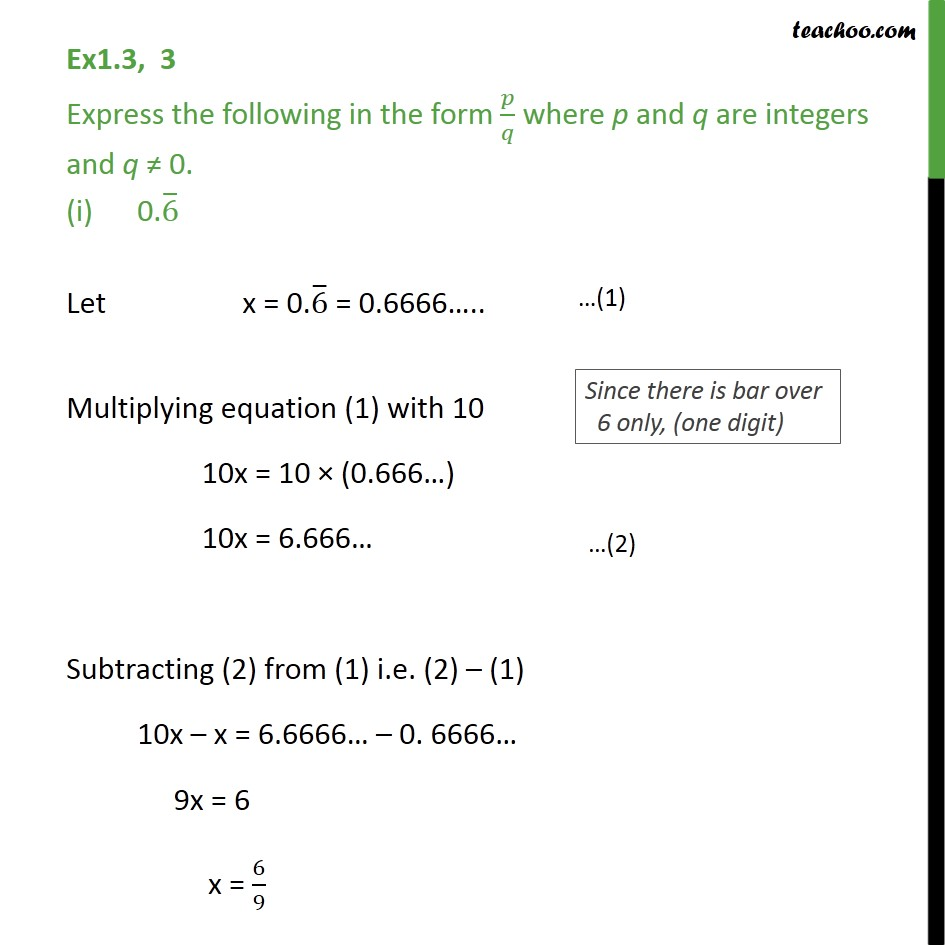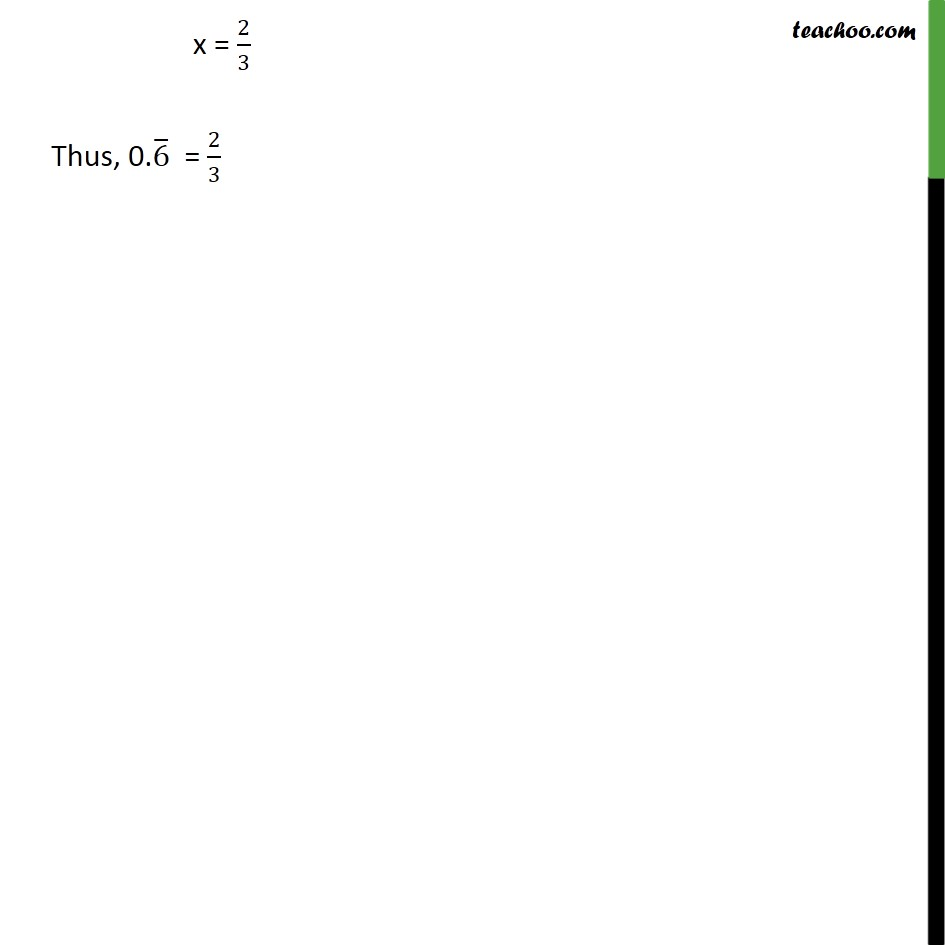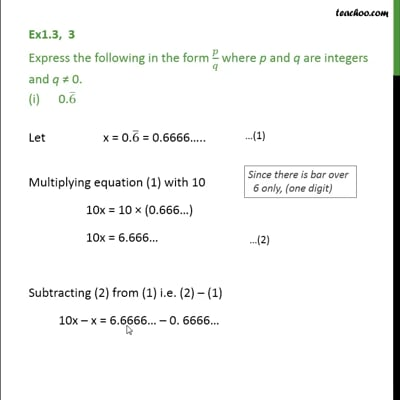Chapter 1 Class 9 Number Systems

Class 9
Important Questions for Exam - Class 9This video is only available for Teachoo black users

### Transcript

Ex1.3, 3 Express the following in the form 𝑝/𝑞 where p and q are integers and q ≠ 0. 0.6 ̅ Let x = 0.6 ̅ = 0.6666….. Multiplying equation (1) with 10 10x = 10 × (0.666…) 10x = 6.666… Subtracting (2) from (1) i.e. (2) – (1) 10x – x = 6.6666… – 0. 6666… 9x = 6 x = 6/9 x = 2/3 Thus, 0.6 ̅ = 2/3